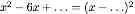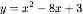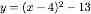## Tuesday, March 25, 2008

### Completing the Square

Toward the goal of sharing more of the humdrum, everyday business of teaching math, here are a few notes about how we do completing the square in my classes. I have no cool tricks or creative activies for this, and I would very much like to see more of yours. Nevertheless, completing the square is, inexplicably, a favorite topic of mine.

The first time I taught it I relied somewhat on the formulaic addition and subtraction of the square of half the middle coefficient, but that left the class utterly confused and frustrated. Now I rely less on this rule and more on pattern recognition and intuition. This appears to make for better retention, though the students do have trouble applying it to numerically messy cases, where a formulaic approach - if ever mastered, that is - would be safer.

We start by reviewing how to square a binomial, because while we have of course worked on multiplying binomials and using standard factoring patterns earlier, the error of squaring a binomial by squaring each term is remarkably resistant to instruction. We always need to refresh that by writing out the factors and multiplying, carefully, term by term. Indeed, any time that a review of completing the square is called for later, squaring binomials from scratch is the point I will return to, and invariably it will turn out that many students have forgotten what the squared binomial looks like. After we've done the multiplication from first principles for a handful of examples, I point out the pattern in the middle and last terms of the product and ask the students to pick up speed, which they do. I write up a few binomials where the second term is a fraction and remind the students that one advantage of fraction form over decimal form is that fractions are really easy to square.

I'll call on individual students or have the class shout out answers, and will alternate between having students suggesting problems and solving them ("J., will you give us a binomial?" "S., will you square that for us?") and I have repeatedly been surprised at how engaged the students tend to become during this exchange, since the topic, after all, isn't that inherently exciting, and we aren't doing anything particularly nifty. Part of the reason may be that it is easier than for many other topics to sense just where the students are and to tailor the next example so that it matches their readiness.

When I notice that the class is beginning to get that "now what...?" feeling, we reverse the process: I write up a perfect square trinomial and have students factor it. We keep doing this until the students again reach the point where this is too easy, and then start looking at cases where only the quadratic and linear terms are given and the students need to figure out what numbers would fit in the blank spaces in a form such as this one:Later, writing this form on the board will be sufficient to cue a large fraction of the students to what they are trying to do.

We move on to rewriting simple quadratics (where a=1 and b is an integer) in vertex form. Later I will show them that adding and subtracting the square of half of the coefficient of the linear term will give us just what we want, but at this stage we simply identify the squared binomial, multiply it out, and compare this with the original quadratic to see what we need to add or subtract. For example, to writein vertex form we will recognize that (x-4)^2 is the square term, and since expanding this gives a constant term of 16 we'll need to subtract 13 in order to ensure that we have the same quadratic that we started with:This approach seems to stick fairly well in students' memories. Many students who do not correctly add and subtract the half of the middle coefficient later (they'll insert an x in there, or halve it incorrectly, or something) will be able to rewrite simple quadratics in vertex form, and I can see from their scratch work in the margin that they're just comparing the expanded square with the original quadratic. I'm pleased with that, because the equivalence of the quadratic in its two forms is one of the big ideas I want them to take away, and the fact that we aren't dealing with different quadratics even though they do look different isn't nearly as self-evident to the young ones as it is to us.

So, that was not terribly exciting or innovative, I concede. But how do you teach completing the square?

## Saturday, March 22, 2008

### Joke

- What's the difference between an outgoing Physicist and one who is not?
- The outgoing Physicist looks at your shoes while talking to you.

Oof.

## Thursday, March 6, 2008

### Pi Day

What do you all do for Pi Day? In particular, what might be worth the while in an Algebra 1 class?

Would anyone with a high-traffic blog mind posting some version of this question, in order to cast a wider net? That would be nice of you...